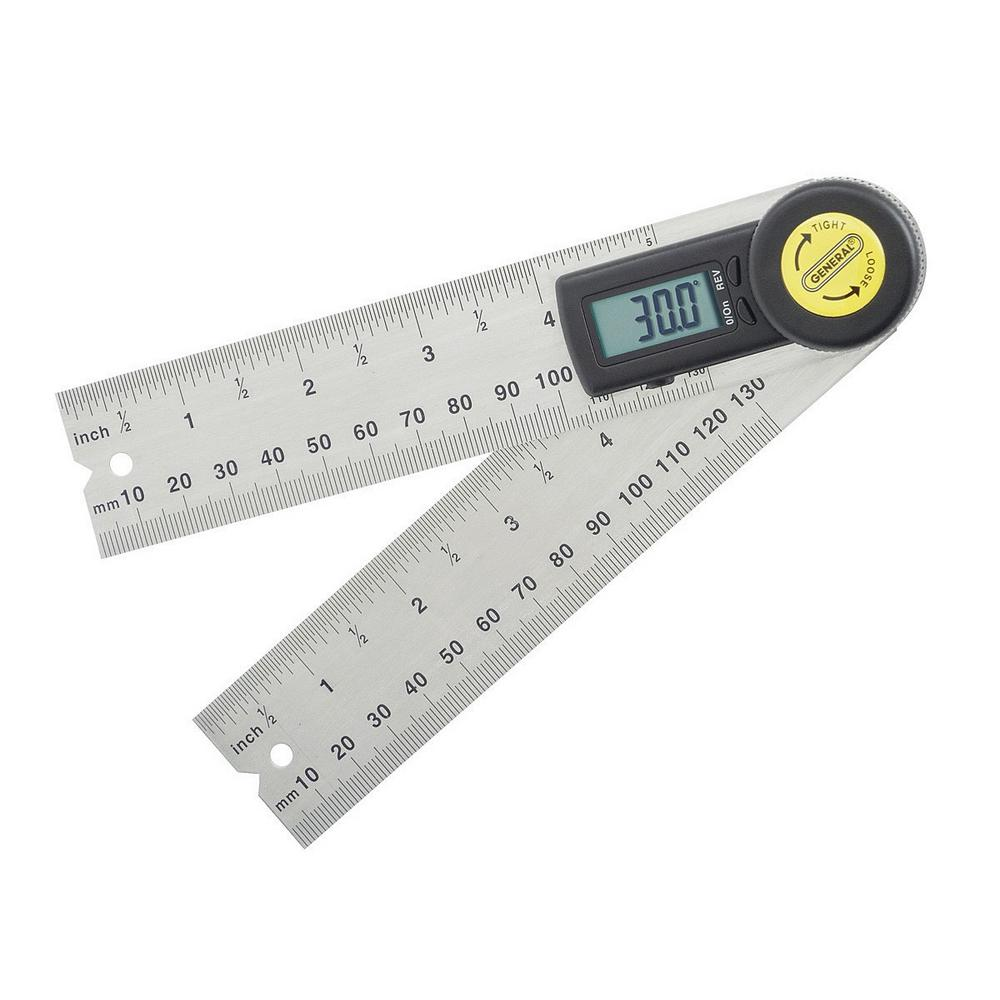## Online Plane Angle Converter ToolType a value in any of the fields to convert between plane angle value:

Details
 Degree A degree, usually denoted by °, is a measurement of a plane angle, defined so that a full rotation is 360 degrees. The gradian is a unit of measurement of an angle, equivalent to of a turn, of a degree, or of a radian. A milliradian, often called a mil or mrad, is an SI derived unit for angular measurement which is defined as a thousandth of a radian. A minute of arc, arcminute, arc minute, or minute arc is a unit of angular measurement equal to of one degree. Since one degree is of a turn, one minute of arc is of a turn. The radian is the SI unit for measuring angles, and is the standard unit of angular measure used in many areas of mathematics. The length of an arc of a unit circle is numerically equal to the measurement in radians of the angle that it subtends. A unit of angular measure equal to 1/60 of an arc minute, or 1/3600 of a degree.